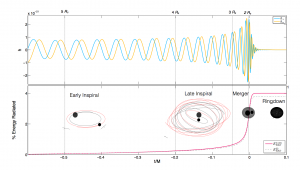# BINARY BLACK HOLE SIMULATIONS

Welcome to the Georgia Tech catalog of binary black hole simulations. The publication describing this catalog can be found on Classical & Quantum Gravity “Georgia tech catalog of gravitational waveforms”

The catalog contains 452 unique simulations. These simulations can be used to analyze the dynamics and data-analysis for gravitational-waves from a binary black hole coalescence. In this site you will find a information about:

1. Table summarizing the binary black hole parameter space of the catalog
2. Gravitational-waveform for a given simulation and relevant scripts
3. Raw simulation data
4. Technical details about the simulations————————-
CATALOG TABLE

The table summarizing the catalog of our simulations (initial parameters of binary black holes and remnant black hole) can be downloaded here

————————-
GRAVITATIONAL WAVEFORMS

These waveform are in LSC-hdf5 format and can be scaled to a given total-mass and distance. The scripts to read these waveforms can be found here.

————————-
TECHNICAL INFORMATION

• In each simulation, binary black holes are scaled to total mass set by the apparent horizon mass at the start of the simulation (ideally, Mtot = 1).
• All gravitational-waveforms (LSC-hdf5 format) contain radiated modes l=2:6, m=-l:l. The waveforms are computed at finite extraction radius and finite resolution. Initial junk radiation is removed from these waveforms.
• Waveforms from multiple extraction radius and individual modes are available in raw simulation data.
• Spins, masses and momenta are defined at the start of the simulation.

————————-
Key Words

GTID := Permanent ID for each simulations
q := mass-ratio of black holes (m1/m2 >=1)
(a1x, a1y, a1z) := dimensionless spin on bigger black hole
(a2x, a2y, a2z) := dimensionless spin on smaller black hole
GT-tag := Internal directory name